# Lower Columbia River Fish Population Indexing Analysis 2018

The suggested citation for this analytic report is:

Thorley, J.L. and Dalgarno, S. (2019) Lower Columbia River Fish Population Indexing Analysis 2018. A Poisson Consulting Analysis Appendix. URL: https://www.poissonconsulting.ca/f/1169613097.

## Background

In the mid 1990s BC Hydro began operating Hugh L. Keenleyside (HLK) Dam to reduce dewatering of Mountain Whitefish and Rainbow Trout eggs.

The primary goal of the Lower Columbia River Fish Population Indexing program is to answer two key management questions:

What are the abundance, growth rate, survival rate, body condition, age distribution, and spatial distribution of subadult and adult Whitefish, Rainbow Trout, and Walleye in the Lower Columbia River?

What is the effect of inter-annual variability in the Whitefish and Rainbow Trout flow regimes on the abundance, growth rate, survival rate, body condition, and spatial distribution of subadult and adult Whitefish, Rainbow Trout, and Walleye in the Lower Columbia River?

The inter-annual variability in the Whitefish and Rainbow Trout flow regimes was quantified in terms of the percent egg dewatering as greater flow variability is associated with more egg stranding.

## Methods

### Data Preparation

The fish indexing data were provided by Okanagan Nation Alliance and Golder Associates in the form of an Access database. The discharge and temperature data were obtained from the Columbia Basin Hydrological Database maintained by Poisson Consulting. The Rainbow Trout egg dewatering estimates were provided by CLBMON-46 (Irvine, Baxter, and Thorley 2015) and the Mountain Whitefish egg stranding estimates by Golder Associates (2013).

#### Discharge

Missing hourly discharge values for Hugh-Keenleyside Dam (HLK), Brilliant Dam (BRD) and Birchbank (BIR) were estimated by first leading the BIR values by 2 hours to account for the lag. Values missing at just one of the dams were then estimated assuming $$HLK + BRD = BIR$$. Negative values were set to be zero. Next, missing values spanning $$\leq$$ 28 days were estimated at HLK and BRD based on linear interpolation. Finally any remaining missing values at BIR were set to be $$HLK + BRD$$.

The data were prepared for analysis using R version 3.6.1 (R Core Team 2018).

### Data Analysis

Model parameters were estimated using hierarchical Bayesian methods. The parameters were produced using JAGS (Plummer 2015) and STAN (Carpenter et al. 2017). For additional information on Bayesian estimation the reader is referred to McElreath (2016).

The one exception is the length-at-age estimates which were produced using the mixdist R package (Macdonald 2012) which implements Maximum Likelihood with Expectation Maximization.

Unless indicated otherwise, the Bayesian analyses used normal and uniform prior distributions that were vague in the sense that they did not constrain the posteriors (Kery and Schaub 2011, 36). The posterior distributions were estimated from 1500 Markov Chain Monte Carlo (MCMC) samples thinned from the second halves of 3 chains (Kery and Schaub 2011, 38–40). Model convergence was confirmed by ensuring that $$\hat{R} \leq 1.05$$ (Kery and Schaub 2011, 40) and $$\textrm{ESS} \geq 150$$ for each of the monitored parameters (Kery and Schaub 2011, 61). Where $$\hat{R}$$ is the potential scale reduction factor and $$\textrm{ESS}$$ is the effective sample size (Brooks et al. 2011).

The parameters are summarised in terms of the point estimate, standard deviation (sd), the z-score, lower and upper 95% confidence/credible limits (CLs) and the p-value (Kery and Schaub 2011, 37, 42). For ML models, the point estimate is the MLE, the standard deviation is the standard error, the z-score is $$\mathrm{MLE}/\mathrm{sd}$$ and the 95% CLs are the $$\mathrm{MLE} \pm 1.96 \cdot \mathrm{sd}$$. For Bayesian models, the estimate is the median (50th percentile) of the MCMC samples, the z-score is $$\mathrm{mean}/\mathrm{sd}$$ and the 95% CLs are the 2.5th and 97.5th percentiles. A p-value of 0.05 indicates that the lower or upper 95% CL is 0.

Where relevant, model adequacy was confirmed by examination of residual plots for the full model(s).

The results are displayed graphically by plotting the modeled relationships between particular variables and the response(s) with the remaining variables held constant. In general, continuous and discrete fixed variables are held constant at their mean and first level values, respectively, while random variables are held constant at their typical values (expected values of the underlying hyperdistributions) (Kery and Schaub 2011, 77–82). When informative the influence of particular variables is expressed in terms of the effect size (i.e., percent change in the response variable) with 95% confidence/credible intervals (CIs, Bradford, Korman, and Higgins 2005).

The analyses were implemented using R version 3.6.1 (R Core Team 2018) and the mbr family of packages.

### Model Descriptions

#### Condition

The expected weight of fish of a given length were estimated from the data using a mass-length model (He et al. 2008). Key assumptions of the condition model include:

• The expected weight is allowed to vary with length and date.
• The expected weight is allowed to vary randomly with year.
• The relationship between weight and length is allowed to vary with date.
• The relationship between weight and length is allowed to vary randomly with year.
• The residual variation in weight is log-normally distributed.

Only previously untagged fish were included in models to avoid potential effects of tagging on body condition. Preliminary analyses indicated that the annual variation in weight was not correlated with the annual variation in the relationship between weight and length.

#### Growth

Annual growth of fish were estimated from the inter-annual recaptures using the Fabens method (Fabens 1965) for estimating the von Bertalanffy growth curve (von Bertalanffy 1938). This curve is based on the premise that:

$\frac{\text{d}L}{\text{d}t} = k (L_{\infty} - L)$

where $$L$$ is the length of the individual, $$k$$ is the growth coefficient and $$L_{\infty}$$ is the maximum length.

Integrating the above equation gives:

$L_t = L_{\infty} (1 - e^{-k(t - t_0)})$

where $$L_t$$ is the length at time $$t$$ and $$t_0$$ is the time at which the individual would have had zero length.

The Fabens form allows

$L_r = L_c + (L_{\infty} - L_c) (1 - e^{-kT})$

where $$L_r$$ is the length at recapture, $$L_c$$ is the length at capture and $$T$$ is the time between capture and recapture.

Key assumptions of the growth model include:

• The mean maximum length $$L_{\infty}$$ is constant.
• The growth coefficient $$k$$ is allowed to vary randomly with year.
• The residual variation in growth is normally distributed.

#### Movement

The extent to which sites are closed, i.e., fish remain at the same site between sessions, was evaluated with a logistic ANCOVA (Kery 2010). The model estimates the probability that intra-annual recaptures were caught at the same site versus a different one. Key assumptions of the site fidelity model include:

• The expected site fidelity is allowed to vary with fish length.
• Observed site fidelity is Bernoulli distributed.

Length as a second-order polynomial was not found to be a significant predictor for site fidelity.

#### Length-At-Age

The expected length-at-age of Mountain Whitefish and Rainbow Trout were estimated from annual length-frequency distributions using a finite mixture distribution model (Macdonald and Pitcher 1979)

There were assumed to be three distinguishable normally-distributed age-classes for Mountain Whitefish (Age-0, Age-1, Age-2 and Age-3+) two for Rainbow Trout (Age-0, Age-1, Age-2+). Initially the model was fitted to the data from all years combined. The model was then fitted to the data for each year separately with the initial values set to be the estimates from the combined values. The only constraints were that the standard deviations of the MW age-classes were identical in the combined analysis and fixed at the initial values in the individual years.

Rainbow Trout and Mountain Whitefish were categorized as Fry (Age-0), Juvenile (Age-1) and Adult (Age-2+) based on their length-based ages. All Walleye were considered to be Adults.

#### Survival

The annual adult survival rate was estimated by fitting a Cormack-Jolly-Seber model (Kery and Schaub 2011, 220–31) to inter-annual recaptures of adults.

Key assumptions of the survival model include:

• Survival varies randomly with year.
• The encounter probability for adults is allowed to vary with the total bank length sampled.

#### Observer Length Correction

The annual bias (inaccuracy) and error (imprecision) in observer’s fish length estimates were quantified from the divergence of the length distribution of their observed fish from the length distribution of the measured fish. More specifically, the length correction that minimised the Jensen-Shannon divergence (Lin 1991) between the two distributions provided a measure of the inaccuracy while the minimum divergence (the Jensen-Shannon divergence was calculated with log to base 2 which means it lies between 0 and 1) provided a measure of the imprecision.

#### Capture Efficiency

The probability of capture was estimated using a recapture-based binomial model (Kery and Schaub 2011, 134–36, 384–88).

Key assumptions of the capture efficiency model include:

• The capture probability varies randomly by session within year.
• The probability of a marked fish remaining at a site is the estimated site fidelity.
• The number of recaptures is described by a binomial distribution.

#### Abundance

The abundance was estimated from the catch and bias-corrected observer count data using an overdispersed Poisson model (Kery and Schaub 2011, 55–56).

Key assumptions of the abundance model include:

• The capture efficiency is the point estimate from the capture efficiency model.
• The efficiency varies by visit type (catch or count).
• The lineal fish density varies randomly with site, year and site within year.
• The overdispersion varies by visit type (count or catch).
• The catches and counts are described by a gamma-Poisson distribution.

#### Stock-Recruitment

The relationship between the adults and the resultant number of age-1 subadults was estimated using a Beverton-Holt stock-recruitment model (Walters and Martell 2004):

$R = \frac{\alpha \cdot S}{1 + \beta \cdot S}$

where $$S$$ is the adults (stock), $$R$$ is the subadults (recruits), $$\alpha$$ is the recruits per spawner at low density (productivity) and $$\beta$$ is the density-dependence.

Key assumptions of the stock-recruitment model include:

• The prior for $$\log(\alpha)$$ is a truncated normal distribution from $$\log(1)$$ to $$\log(5)$$.
• The expected log number of recruits is affected by the egg loss.
• The residual variation in the number of recruits is log-normally distributed.

#### Age-Ratios

The proportion of Age-1 Mountain Whitefish $$r^1_t$$ from a given spawn year $$t$$ is calculated from the relative abundance of Age-1 & Age-2 fish $$N^1_t$$ & $$N^2_t$$ respectively, which were lead or lagged so that all values were with respect to the spawn year:

$r^1_t = \frac{N^1_{t+2}}{N^1_{t+2} + N^2_{t+2}}$

The relative abundances of Age-1 and Age-2 fish were taken from the proportions of each age-class in the length-at-age analysis.

As the number of Age-2 fish might be expected to be influenced by the percentage egg loss $$Q_t$$ three years prior, the predictor variable $$\Pi_t$$ used is:

$\Pi_t = \textrm{log}(Q_t/Q_{t-1})$

The ratio was logged to ensure it was symmetrical about zero (Tornqvist, Vartia, and Vartia 1985).

The relationship between $$r^1_t$$ and $$\Pi_t$$ was estimated using a Bayesian regression (Kery 2010) loss model.

Key assumptions of the final model include:

• The log odds of the proportion of Age-1 fish varies with the log of the ratio of the percent egg losses.
• The residual variation is normally distributed.

The relationship between percent dewatering and subsequent recruitment is expected to depend on stock abundance (Subbey et al. 2014) which might be changing over the course of the study. Consequently, preliminary analyses allowed the slope of the regression line to change by year. However, year was not a significant predictor and was therefore removed from the final model. The effect of dewatering on Mountain Whitefish abundance was expressed in terms of the predicted percent change in Age-1 Mountain Whitefish abundance by egg loss in the spawn year relative to 10% egg loss in the spawn year. The egg loss in the previous year was fixed at 10%. The percent change could not be calculated relative to 0% in the spawn or previous year as $$\Pi_t$$ is undefined in either case.

### Model Templates

#### Condition

 data {
int nYear;
int nObs;

vector[nObs] Length;
vector[nObs] Weight;
vector[nObs] Dayte;
int Year[nObs];

parameters {
real bWeight;
real bWeightLength;
real bWeightDayte;
real bWeightLengthDayte;
real sWeightYear;
real sWeightLengthYear;

vector[nYear] bWeightYear;
vector[nYear] bWeightLengthYear;
real sWeight;

model {

vector[nObs] eWeight;

bWeight ~ normal(5, 5);
bWeightLength ~ normal(3, 2);

bWeightDayte ~ normal(0, 2);
bWeightLengthDayte ~ normal(0, 2);

sWeightYear ~ normal(0, 2);
sWeightLengthYear ~ normal(0, 2);

for (i in 1:nYear) {
bWeightYear[i] ~ normal(0, exp(sWeightYear));
bWeightLengthYear[i] ~ normal(0, exp(sWeightLengthYear));
}

sWeight ~ normal(0, 5);
for(i in 1:nObs) {
eWeight[i] = bWeight + bWeightDayte * Dayte[i] + bWeightYear[Year[i]] + (bWeightLength + bWeightLengthDayte * Dayte[i] + bWeightLengthYear[Year[i]]) * Length[i];
Weight[i] ~ lognormal(eWeight[i], exp(sWeight));
}
..

Block 1.

#### Growth

.model {
bK ~ dnorm (0, 5^-2)
sKYear ~ dnorm(0, 5^-2)

for (i in 1:nYear) {
bKYear[i] ~ dnorm(0, exp(sKYear)^-2)
log(eK[i]) <- bK + bKYear[i]
}

bLinf ~ dunif(100, 1000)
sGrowth ~ dnorm(0, 5^-2)
for (i in 1:length(Year)) {
eGrowth[i] <- (bLinf - LengthAtRelease[i]) * (1 - exp(-sum(eK[Year[i]:(Year[i] + dYears[i] - 1)])))
Growth[i] ~ dnorm(max(eGrowth[i], 0), exp(sGrowth)^-2)
}
..

Block 2.

#### Movement

.model {

bFidelity ~ dnorm(0, 2^-2)
bLength ~ dnorm(0, 2^-2)

for (i in 1:length(Fidelity)) {
logit(eFidelity[i]) <- bFidelity + bLength * Length[i]
Fidelity[i] ~ dbern(eFidelity[i])
}
..

Block 3.

#### Survival

.model{
bEfficiency ~ dnorm(0, 5^-2)
bEfficiencySampledLength ~ dnorm(0, 5^-2)

bSurvival ~ dnorm(0, 5^-2)

sSurvivalYear ~ dnorm(0, 5^-2)
for(i in 1:nYear) {
bSurvivalYear[i] ~ dnorm(0, exp(sSurvivalYear)^-2)
}

for(i in 1:(nYear-1)) {
logit(eEfficiency[i]) <- bEfficiency + bEfficiencySampledLength * SampledLength[i]
logit(eSurvival[i]) <- bSurvival + bSurvivalYear[i]

eProbability[i,i] <- eSurvival[i] * eEfficiency[i]
for(j in (i+1):(nYear-1)) {
eProbability[i,j] <- prod(eSurvival[i:j]) * prod(1-eEfficiency[i:(j-1)]) * eEfficiency[j]
}
for(j in 1:(i-1)) {
eProbability[i,j] <- 0
}
}
for(i in 1:(nYear-1)) {
eProbability[i,nYear] <- 1 - sum(eProbability[i,1:(nYear-1)])
}

for(i in 1:(nYear - 1)) {
Marray[i, 1:nYear] ~ dmulti(eProbability[i,], Released[i])
}
..

Block 4.

#### Capture Efficiency

.model {

bEfficiency ~ dnorm(0, 5^-2)

sEfficiencySessionAnnual ~ dnorm(0, 2^-2)
for (i in 1:nSession) {
for (j in 1:nAnnual) {
bEfficiencySessionAnnual[i, j] ~ dnorm(0, exp(sEfficiencySessionAnnual)^-2)
}
}

for (i in 1:length(Recaptures)) {

logit(eEfficiency[i]) <- bEfficiency + bEfficiencySessionAnnual[Session[i], Annual[i]]

eFidelity[i] ~ dnorm(Fidelity[i], FidelitySD[i]^-2) T(FidelityLower[i], FidelityUpper[i])
Recaptures[i] ~ dbin(eEfficiency[i] * eFidelity[i], Tagged[i])
}
..

Block 5.

#### Abundance

.model {
bDensity ~ dnorm(5, 5^-2)

sDensityAnnual ~ dnorm(0, 2^-2)
for (i in 1:nAnnual) {
bDensityAnnual[i] ~ dnorm(0, exp(sDensityAnnual)^-2)
}

sDensitySite ~ dnorm(0, 2^-2)
sDensitySiteAnnual ~ dnorm(0, 2^-2)
for (i in 1:nSite) {
bDensitySite[i] ~ dnorm(0, exp(sDensitySite)^-2)
for (j in 1:nAnnual) {
bDensitySiteAnnual[i, j] ~ dnorm(0, exp(sDensitySiteAnnual)^-2)
}
}

bEfficiencyVisitType <- 0
for (i in 2:nVisitType) {
bEfficiencyVisitType[i] ~ dnorm(0, 2^-2)
}

sDispersion ~ dnorm(0, 2^-2)
sDispersionVisitType <- 0
for(i in 2:nVisitType) {
sDispersionVisitType[i] ~ dnorm(0, 2^-2)
}

for (i in 1:length(Fish)) {
log(eDensity[i]) <- bDensity + bDensitySite[Site[i]] + bDensityAnnual[Annual[i]] + bDensitySiteAnnual[Site[i],Annual[i]]

eAbundance[i] <- eDensity[i] * SiteLength[i]

logit(eEfficiency[i]) <- logit(Efficiency[i]) + bEfficiencyVisitType[VisitType[i]]

log(esDispersion[i]) <- sDispersion + sDispersionVisitType[VisitType[i]]

eDispersion[i] ~ dgamma(esDispersion[i]^-2 + 0.1, esDispersion[i]^-2 + 0.1)
eFish[i] <- eAbundance[i] * ProportionSampled[i] * eEfficiency[i]
Fish[i] ~ dpois(eFish[i] * eDispersion[i])
}
..

Block 6.

#### Stock-Recruitment

.model {

bAlpha ~ dnorm(1, 2^-2) T(log(1), log(5))
bBeta ~ dnorm(-10, 5^-2)
bEggLoss ~ dnorm(0, 2^-2)

sRecruits ~ dnorm(0, 1^-2) T(0,)
for(i in 1:length(Stock)){
log(eAlpha[i]) <- bAlpha
log(eBeta[i]) <- bBeta
eLogRecruits[i] <- log((eAlpha[i] * Stock[i]) / (1 + eBeta[i] * Stock[i])) + bEggLoss * EggLoss[i]
Recruits[i] ~ dlnorm(eLogRecruits[i], sRecruits^-2)
}
..

Block 7.

#### Age-Ratios

.model{
bProbAge1 ~ dnorm(0, 2^-2)
bProbAge1Loss ~ dnorm(0, 2^-2)

sProbAge1 ~ dunif(0, 2)
for(i in 1:length(Age1Prop)){
eAge1Prop[i] <- bProbAge1 + bProbAge1Loss * LossLogRatio[i]
Age1Prop[i] ~ dnorm(eAge1Prop[i], sProbAge1^-2)
}
..

Block 8.

## Results

### Tables

#### Condition

Table 1. Parameter descriptions.

Parameter Description
bWeight Intercept of log(eWeight)
bWeightDayte Effect of Dayte on bWeight
bWeightLength Intercept of effect of Length on bWeight
bWeightLengthDayte Effect of Dayte on bWeightLength
bWeightLengthYear[i] Effect of ith Year on bWeightLength
bWeightYear[i] Effect of ith Year on bWeight
Dayte[i] Standardised day of year ith fish was captured
eWeight[i] Expected Weight of ith fish
Length[i] Log-transformed and centered fork length of ith fish
sWeight Log standard deviation of residual variation in log(Weight)
sWeightLengthYear Log standard deviation of bWeightLengthYear
sWeightYear Log standard deviation of bWeightYear
Weight[i] Recorded weight of ith fish
Year[i] Year ith fish was captured
##### Mountain Whitefish

Table 2. Model coefficients.

term estimate sd zscore lower upper pvalue
bWeight 5.4481708 0.0099010 550.217864 5.4277038 5.4674031 0.0007
bWeightDayte -0.0176867 0.0018633 -9.517441 -0.0213734 -0.0141445 0.0007
bWeightLength 3.1579282 0.0233766 135.080079 3.1093590 3.2040653 0.0007
bWeightLengthDayte -0.0122878 0.0050029 -2.457204 -0.0221361 -0.0026500 0.0093
sWeight -1.9095091 0.0060218 -317.076006 -1.9208347 -1.8977600 0.0007
sWeightLengthYear -2.2657732 0.1913599 -11.805313 -2.6206595 -1.8801177 0.0007
sWeightYear -3.1215164 0.1734585 -17.942536 -3.4339287 -2.7488788 0.0007

Table 3. Model summary.

n K nchains niters nthin ess rhat converged
14178 7 3 500 2 393 1.011 TRUE
##### Rainbow Trout

Table 4. Model coefficients.

term estimate sd zscore lower upper pvalue
bWeight 5.9948771 0.0062049 966.160917 5.9819110 6.0072682 7e-04
bWeightDayte -0.0044249 0.0012834 -3.475668 -0.0069787 -0.0019914 7e-04
bWeightLength 2.9215460 0.0125398 232.960088 2.8962627 2.9453204 7e-04
bWeightLengthDayte 0.0377059 0.0038700 9.744408 0.0301796 0.0451479 7e-04
sWeight -2.2655650 0.0058125 -389.785952 -2.2774300 -2.2545811 7e-04
sWeightLengthYear -2.9060607 0.1892851 -15.342687 -3.2594914 -2.5229922 7e-04
sWeightYear -3.6317633 0.1684327 -21.507633 -3.9415447 -3.2894396 7e-04

Table 5. Model summary.

n K nchains niters nthin ess rhat converged
14589 7 3 500 2 374 1.009 TRUE
##### Walleye

Table 6. Model coefficients.

term estimate sd zscore lower upper pvalue
bWeight 6.2919286 0.0080132 785.139922 6.2739150 6.3064459 0.0007
bWeightDayte 0.0161593 0.0014148 11.389367 0.0134151 0.0188725 0.0007
bWeightLength 3.2304215 0.0191397 168.779806 3.1904598 3.2675943 0.0007
bWeightLengthDayte -0.0107375 0.0083310 -1.277545 -0.0272052 0.0058224 0.1947
sWeight -2.3681131 0.0073079 -324.012600 -2.3819666 -2.3535583 0.0007
sWeightLengthYear -2.5323101 0.1992700 -12.680344 -2.8975376 -2.1164227 0.0007
sWeightYear -3.3305458 0.1668623 -19.908489 -3.6302314 -2.9748845 0.0007

Table 7. Model summary.

n K nchains niters nthin ess rhat converged
9205 7 3 500 2 393 1.014 TRUE

#### Growth

Table 8. Parameter descriptions.

Parameter Description
bK Intercept of log(eK)
bKYear[i] Effect of ith Year on bK
bLinf Mean maximum length
dYears[i] Years between release and recapture of ith recapture
eGrowth Expected Growth between release and recapture
eK[i] Expected von Bertalanffy growth coefficient from i-1th to ith year
Growth[i] Observed growth between release and recapture of ith recapture
LengthAtRelease[i] Length at previous release of ith recapture
sGrowth Log standard deviation of residual variation in Growth
sKYear Log standard deviation of bKYear
Year[i] Release year of ith recapture
##### Mountain Whitefish

Table 9. Model coefficients.

term estimate sd zscore lower upper pvalue
bK -0.9259595 0.1086078 -8.559326 -1.149029 -0.7297757 7e-04
bLinf 392.6903086 3.2148232 122.202222 386.888094 399.3993119 7e-04
sGrowth 2.4601904 0.0443263 55.511488 2.377194 2.5493541 7e-04
sKYear -1.0848023 0.2570075 -4.222173 -1.557970 -0.5837363 7e-04

Table 10. Model summary.

n K nchains niters nthin ess rhat converged
259 4 3 500 20 543 1.013 TRUE
##### Rainbow Trout

Table 11. Model coefficients.

term estimate sd zscore lower upper pvalue
bK -0.1744708 0.0762919 -2.306990 -0.3307308 -0.0225079 2e-02
bLinf 487.0687845 2.7075093 179.890601 481.9086684 492.4080715 7e-04
sGrowth 3.3811271 0.0206856 163.459867 3.3411191 3.4218987 7e-04
sKYear -1.2416744 0.1924957 -6.405807 -1.5677043 -0.8305064 7e-04

Table 12. Model summary.

n K nchains niters nthin ess rhat converged
1158 4 3 500 20 400 1.011 TRUE
##### Walleye

Table 13. Model coefficients.

term estimate sd zscore lower upper pvalue
bK -2.539502 0.2502549 -10.147884 -3.001751 -2.0618755 7e-04
bLinf 754.315450 84.3008026 9.083864 628.631688 949.4953241 7e-04
sGrowth 2.866226 0.0460119 62.295651 2.778109 2.9608715 7e-04
sKYear -1.157805 0.2545813 -4.530802 -1.636968 -0.6447953 7e-04

Table 14. Model summary.

n K nchains niters nthin ess rhat converged
263 4 3 500 40 222 1.006 TRUE

#### Movement

Table 15. Parameter descriptions.

Parameter Description
bFidelity Intercept of logit(eFidelity)
bLength Effect of length on logit(eFidelity)
eFidelity[i] Expected site fidelity of ith recapture
Fidelity[i] Whether the ith recapture was encountered at the same site as the previous encounter
Length[i] Length at previous encounter of ith recapture
##### Mountain Whitefish

Table 16. Model coefficients.

term estimate sd zscore lower upper pvalue
bFidelity -0.1552880 0.1792936 -0.8567948 -0.4959377 0.1981948 0.388
bLength -0.1105809 0.1946158 -0.5319073 -0.4760564 0.2944789 0.592

Table 17. Model summary.

n K nchains niters nthin ess rhat converged
117 2 3 500 1 847 1.002 TRUE
##### Rainbow Trout

Table 18. Model coefficients.

term estimate sd zscore lower upper pvalue
bFidelity 0.7654122 0.0796299 9.626364 0.6143531 0.9178480 7e-04
bLength -0.3300007 0.0769163 -4.272205 -0.4739996 -0.1766411 7e-04

Table 19. Model summary.

n K nchains niters nthin ess rhat converged
756 2 3 500 1 774 1.001 TRUE
##### Walleye

Table 20. Model coefficients.

term estimate sd zscore lower upper pvalue
bFidelity 0.7258183 0.1486066 4.9023664 0.4342957 1.0092428 0.0007
bLength -0.0188939 0.1390122 -0.1504418 -0.2987952 0.2483826 0.8800

Table 21. Model summary.

n K nchains niters nthin ess rhat converged
220 2 3 500 1 942 1.003 TRUE

#### Length-At-Age

##### Mountain Whitefish

Table 22. The estimated upper length cutoffs (mm) by age and year.

Year Age0 Age1 Age2
1990 163 275 NA
1991 144 226 296
2001 141 257 344
2002 163 260 343
2003 159 263 353
2004 158 249 342
2005 168 263 362
2006 175 284 357
2007 171 279 337
2008 170 248 341
2009 169 265 355
2010 177 272 353
2011 163 269 349
2012 162 268 347
2013 185 282 350
2014 178 283 362
2015 167 278 366
2016 165 283 352
2017 158 269 354
2018 177 262 346
##### Rainbow Trout

Table 23. The estimated upper length cutoffs (mm) by age and year.

Year Age0 Age1
1990 155 358
1991 127 342
2001 133 324
2002 155 349
2003 161 342
2004 142 332
2005 164 346
2006 170 364
2007 166 375
2008 146 339
2009 147 338
2010 143 337
2011 156 343
2012 152 344
2013 169 354
2014 154 337
2015 167 334
2016 154 336
2017 133 316
2018 139 306

#### Survival

Table 24. Parameter descriptions.

Parameter Description
bEfficiency Intercept for logit(eEfficiency)
bEfficiencySampledLength Effect of SampledLength on bEfficiency
bSurvival Intercept for logit(eSurvival)
bSurvivalYear[i] Effect of Year on bSurvival
eEfficiency[i] Expected recapture probability in ith year
eSurvival[i] Expected survival probability from i-1th to ith year
SampledLength Total standardised length of river sampled
sSurvivalYear Log SD of bSurvivalYear
##### Mountain Whitefish

Table 25. Model coefficients.

term estimate sd zscore lower upper pvalue
bEfficiency -4.1804013 0.1042621 -40.087541 -4.3845229 -3.9700036 0.0007
bEfficiencySampledLength 0.3721012 0.1235324 3.044773 0.1361591 0.6260196 0.0027
bSurvival 0.8463913 0.4231537 2.100816 0.1262261 1.8068358 0.0253
sSurvivalYear 0.2442526 0.3452415 0.739245 -0.4118839 0.9193125 0.4587

Table 26. Model summary.

n K nchains niters nthin ess rhat converged
17 4 3 500 100 1052 1.005 TRUE
##### Rainbow Trout

Table 27. Model coefficients.

term estimate sd zscore lower upper pvalue
bEfficiency -2.5293162 0.0910345 -27.7736502 -2.7044300 -2.3480069 0.0007
bEfficiencySampledLength 0.0111727 0.0715640 0.1401614 -0.1288650 0.1565815 0.8907
bSurvival -0.4348034 0.1118249 -3.8860616 -0.6559384 -0.2061208 0.0007
sSurvivalYear -1.2570805 1.0270840 -1.4226367 -4.9922516 -0.6119388 0.0007

Table 28. Model summary.

n K nchains niters nthin ess rhat converged
17 4 3 500 100 261 1.017 TRUE
##### Walleye

Table 29. Model coefficients.

term estimate sd zscore lower upper pvalue
bEfficiency -3.3757879 0.1030395 -32.8019436 -3.5811971 -3.1708020 0.0007
bEfficiencySampledLength 0.0587105 0.0826296 0.7621854 -0.0929374 0.2316543 0.4440
bSurvival 0.0628850 0.1358752 0.5602158 -0.1683843 0.3885211 0.5440
sSurvivalYear -1.0997005 1.9896128 -0.9160875 -8.2747079 -0.2785067 0.0040

Table 30. Model summary.

n K nchains niters nthin ess rhat converged
17 4 3 500 200 285 1.012 TRUE

#### Capture Efficiency

Table 31. Parameter descriptions.

Parameter Description
Annual[i] Year of ith visit
bEfficiency Intercept for logit(eEfficiency)
bEfficiencySessionAnnual Effect of Session within Annual on logit(eEfficiency)
eEfficiency[i] Expected efficiency on ith visit
eFidelity[i] Expected site fidelity on ith visit
Fidelity[i] Mean site fidelity on ith visit
FidelitySD[i] SD of site fidelity on ith visit
Recaptures[i] Number of marked fish recaught during ith visit
sEfficiencySessionAnnual Log SD of effect of Session within Annual on logit(eEfficiency)
Session[i] Session of ith visit
Tagged[i] Number of marked fish tagged prior to ith visit
##### Mountain Whitefish

Table 32. Model coefficients.

term estimate sd zscore lower upper pvalue
bEfficiency -4.9972025 0.2168087 -23.144160 -5.50063 -4.6570936 0.0007
sEfficiencySessionAnnual -0.9461967 1.1534489 -1.110064 -4.39114 0.0966403 0.0907

Table 33. Model summary.

n K nchains niters nthin ess rhat converged
1359 2 3 500 100 172 1.011 TRUE

Table 34. Model coefficients.

term estimate sd zscore lower upper pvalue
bEfficiency -5.254932 0.1430929 -36.755286 -5.550742 -4.9969882 7e-04
sEfficiencySessionAnnual -2.049795 1.1473121 -1.939951 -5.029096 -0.5983976 7e-04

Table 35. Model summary.

n K nchains niters nthin ess rhat converged
1545 2 3 500 200 356 1.01 TRUE
##### Rainbow Trout

Table 36. Model coefficients.

term estimate sd zscore lower upper pvalue
bEfficiency -3.3923810 0.0654085 -51.880249 -3.524317 -3.2695877 7e-04
sEfficiencySessionAnnual -0.9259046 0.1647731 -5.624789 -1.263903 -0.6153031 7e-04

Table 37. Model summary.

n K nchains niters nthin ess rhat converged
1558 2 3 500 100 1214 1.005 TRUE

Table 38. Model coefficients.

term estimate sd zscore lower upper pvalue
bEfficiency -4.019309 0.0710880 -56.58399 -4.162507 -3.8854350 7e-04
sEfficiencySessionAnnual -1.805271 0.8881719 -2.28053 -4.395410 -0.9157114 7e-04

Table 39. Model summary.

n K nchains niters nthin ess rhat converged
1629 2 3 500 100 244 1.009 TRUE
##### Walleye

Table 40. Model coefficients.

term estimate sd zscore lower upper pvalue
bEfficiency -4.5702137 0.1218943 -37.523799 -4.8186384 -4.3526356 0.0007
sEfficiencySessionAnnual -0.5577893 0.2079586 -2.704928 -0.9992889 -0.1707303 0.0013

Table 41. Model summary.

n K nchains niters nthin ess rhat converged
1673 2 3 500 100 1191 1.004 TRUE

#### Abundance

Table 42. Parameter descriptions.

Parameter Description
Annual Year
bDensity Intercept for log(eDensity)
bDensityAnnual Effect of Annual on bDensity
bDensitySite Effect of Site on bDensity
bDensitySiteAnnual Effect of Site within Annual on bDensity
bEfficiencyVisitType Effect of VisitType on Efficiency
eDensity Expected density
Efficiency Capture efficiency
esDispersion Overdispersion of Fish
Fish Number of fish captured or counted
ProportionSampled Proportion of site surveyed
sDensityAnnual Log SD of effect of Annual on bDensity
sDensitySite Log SD of effect of Site on bDensity
sDensitySiteAnnual Log SD of effect of Site within Annual on bDensity
sDispersion Intercept for log(esDispersion)
sDispersionVisitType Effect of VisitType on sDispersion
Site Site
SiteLength Length of site
VisitType Survey type (catch versus count)
##### Mountain Whitefish

Table 43. Model coefficients.

term estimate sd zscore lower upper pvalue
bDensity 5.4406580 0.1874181 29.013274 5.0405099 5.7930340 0.0007
bEfficiencyVisitType 1.4556995 0.0806910 18.040699 1.2955386 1.6214112 0.0007
sDensityAnnual -0.3775397 0.1736743 -2.125087 -0.6879389 -0.0297508 0.0387
sDensitySite -0.2804839 0.1087247 -2.544630 -0.4863189 -0.0637620 0.0120
sDensitySiteAnnual -0.8244691 0.0611086 -13.458192 -0.9419095 -0.7030915 0.0007
sDispersion -0.7661634 0.0449215 -17.069776 -0.8547301 -0.6842493 0.0007
sDispersionVisitType 0.6067664 0.0927844 6.551051 0.4258833 0.7808791 0.0007

Table 44. Model summary.

n K nchains niters nthin ess rhat converged
2551 7 3 500 200 242 1.009 TRUE

Table 45. Model coefficients.

term estimate sd zscore lower upper pvalue
bDensity 6.3926287 0.1626779 39.271714 6.0770985 6.7111804 0.0007
bEfficiencyVisitType 1.7286939 0.0804946 21.492578 1.5693144 1.8887179 0.0007
sDensityAnnual -1.0772117 0.1991107 -5.379547 -1.4486766 -0.6795237 0.0007
sDensitySite 0.1034875 0.0951208 1.059008 -0.0796085 0.2881605 0.2853
sDensitySiteAnnual -0.9089343 0.0653335 -13.926549 -1.0397152 -0.7879970 0.0007
sDispersion -0.6528188 0.0346453 -18.859430 -0.7171653 -0.5855589 0.0007
sDispersionVisitType 0.4805907 0.0803911 5.973736 0.3199828 0.6406288 0.0007

Table 46. Model summary.

n K nchains niters nthin ess rhat converged
2551 7 3 500 200 285 1.008 TRUE
##### Rainbow Trout

Table 47. Model coefficients.

term estimate sd zscore lower upper pvalue
bDensity 4.8366559 0.1161441 41.651809 4.6119463 5.0539153 7e-04
bEfficiencyVisitType 1.4215737 0.0776402 18.346412 1.2804333 1.5847675 7e-04
sDensityAnnual -1.1758941 0.2012193 -5.774457 -1.5226979 -0.7263475 7e-04
sDensitySite -0.3802453 0.0972301 -3.875574 -0.5617739 -0.1774587 7e-04
sDensitySiteAnnual -0.8930931 0.0557025 -16.052414 -1.0040788 -0.7882411 7e-04
sDispersion -0.9650807 0.0398708 -24.228399 -1.0473340 -0.8914979 7e-04
sDispersionVisitType 0.6569666 0.0897573 7.266721 0.4830828 0.8315600 7e-04

Table 48. Model summary.

n K nchains niters nthin ess rhat converged
2551 7 3 500 200 494 1.008 TRUE

Table 49. Model coefficients.

term estimate sd zscore lower upper pvalue
bDensity 5.4942462 0.1140338 48.164683 5.2728280 5.7120537 7e-04
bEfficiencyVisitType 1.2869357 0.0602682 21.380955 1.1664900 1.4055163 7e-04
sDensityAnnual -1.0927513 0.1848807 -5.862344 -1.4319721 -0.7008315 7e-04
sDensitySite -0.4651834 0.0931610 -4.958562 -0.6378218 -0.2822007 7e-04
sDensitySiteAnnual -1.2089195 0.0693396 -17.453081 -1.3503149 -1.0796723 7e-04
sDispersion -1.0166974 0.0437112 -23.290154 -1.1051124 -0.9356607 7e-04
sDispersionVisitType 0.5491976 0.0927775 5.949737 0.3708907 0.7335595 7e-04

Table 50. Model summary.

n K nchains niters nthin ess rhat converged
2551 7 3 500 200 538 1.006 TRUE
##### Walleye

Table 51. Model coefficients.

term estimate sd zscore lower upper pvalue
bDensity 5.4321978 0.1158070 46.940936 5.2043312 5.6606843 7e-04
bEfficiencyVisitType 1.1735932 0.0756155 15.543691 1.0203961 1.3285330 7e-04
sDensityAnnual -0.8466866 0.1806938 -4.642299 -1.1679495 -0.4744504 7e-04
sDensitySite -1.0459901 0.1388762 -7.514605 -1.3137513 -0.7765578 7e-04
sDensitySiteAnnual -1.3244656 0.0846409 -15.702992 -1.5117795 -1.1702710 7e-04
sDispersion -0.8224011 0.0390031 -21.050567 -0.8956316 -0.7446447 7e-04
sDispersionVisitType 0.5073740 0.0931255 5.432866 0.3154596 0.6789489 7e-04

Table 52. Model summary.

n K nchains niters nthin ess rhat converged
2551 7 3 500 200 682 1.008 TRUE

#### Stock-Recruitment

Table 53. Parameter descriptions.

Parameter Description
bAlpha Intercept for log(eAlpha)
bBeta Intercept for log(eBeta)
bEggLoss Effect of EggLoss on bBeta
eAlpha eRecruits per Stock at low Stock density
eBeta Expected density-dependence
EggLoss Calculated proportional egg loss
eRecruits Expected Recruits
Recruits Number of Age-1 recruits
sRecruits Log SD of residual variation in Recruits
Stock Number of Age-2+ spawners
##### Mountain Whitefish

Table 54. Model coefficients.

term estimate sd zscore lower upper pvalue
bAlpha 0.9003438 0.4528069 1.9298312 0.0575934 1.5750652 0.0007
bBeta -9.6517720 0.5478618 -17.7067921 -10.7832662 -8.8115021 0.0007
bEggLoss -0.1312203 0.1693046 -0.7867717 -0.4716888 0.2092525 0.4187
sRecruits 0.6146288 0.1334134 4.7609870 0.4290761 0.9387169 0.0007

Table 55. Model summary.

n K nchains niters nthin ess rhat converged
16 4 3 500 50 1262 1.002 TRUE
##### Rainbow Trout

Table 56. Model coefficients.

term estimate sd zscore lower upper pvalue
bAlpha 1.0563379 0.3949465 2.567620 0.1819702 1.5837393 0.0007
bBeta -9.1223234 0.5321613 -17.294711 -10.4142573 -8.4729122 0.0007
bEggLoss 0.1661576 0.0742890 2.248995 0.0252849 0.3241453 0.0280
sRecruits 0.2746352 0.0594218 4.793799 0.1976847 0.4334546 0.0007

Table 57. Model summary.

n K nchains niters nthin ess rhat converged
17 4 3 500 50 532 1.006 TRUE

#### Age-Ratios

Table 58. Parameter descriptions.

Parameter Description
Age1[i] The number of Age-1 fish in the ith year
Age1and2[i] The number of Age-1 and Age-2 fish in the ith year
bProbAge1 Intercept for logit(eProbAge1)
bProbAge1Loss Effect of LossLogRatio on bProbAge1
eProbAge1[i] The expected proportion of Age-1 fish in the ith year
LossLogRatio[i] The log of the ratio of the percent egg losses
sDispersion SD of extra-binomial variation

Table 59. Model coefficients.

term estimate sd zscore lower upper pvalue
bProbAge1 0.2409373 0.2021096 1.155502 -0.1779642 0.6160426 0.2400
bProbAge1Loss -0.3070399 0.2870403 -1.052766 -0.8735155 0.2271430 0.2920
sProbAge1 0.8203105 0.1706548 4.943255 0.5839656 1.2571520 0.0007

Table 60. Model summary.

n K nchains niters nthin ess rhat converged
18 3 3 500 1 668 1.006 TRUE

### Figures

#### Condition

###### Mountain Whitefish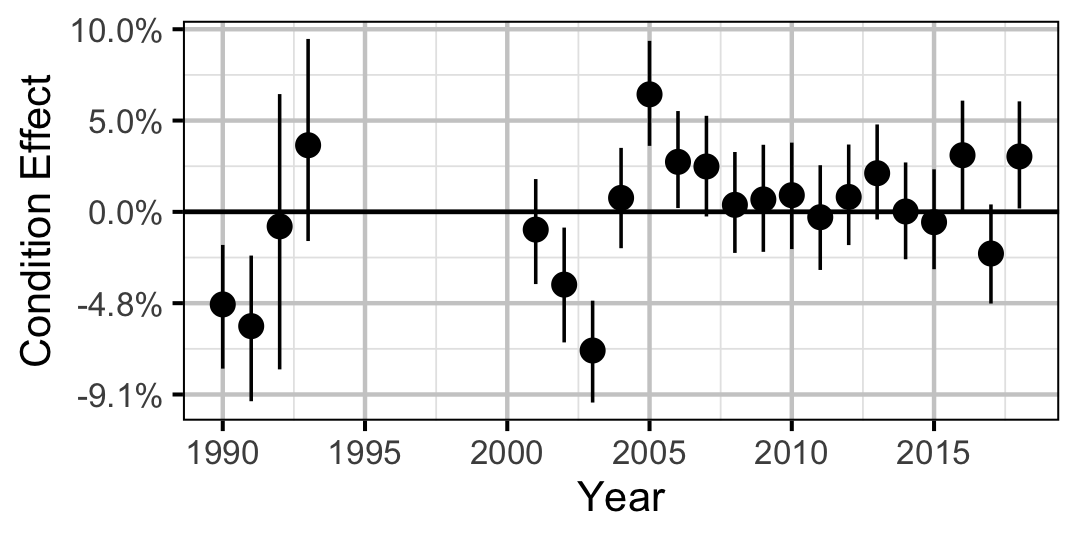Figure 2. Estimated change in condition relative to a typical year for a 200 mm Mountain Whitefish by year (with 95% CRIs).
###### Rainbow Trout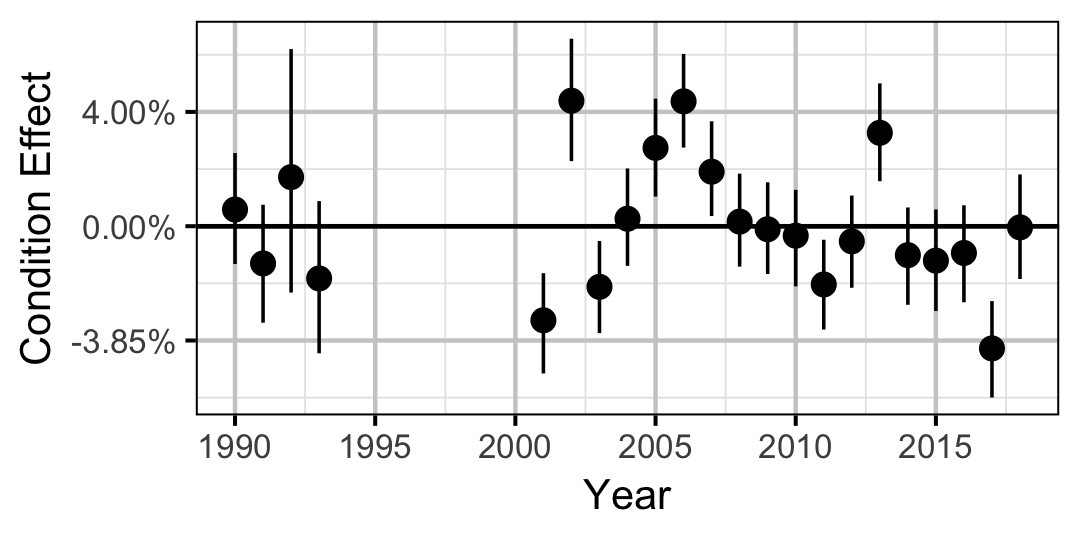Figure 3. Estimated change in condition relative to a typical year for a 250 mm Rainbow Trout by year (with 95% CRIs).
###### Mountain Whitefish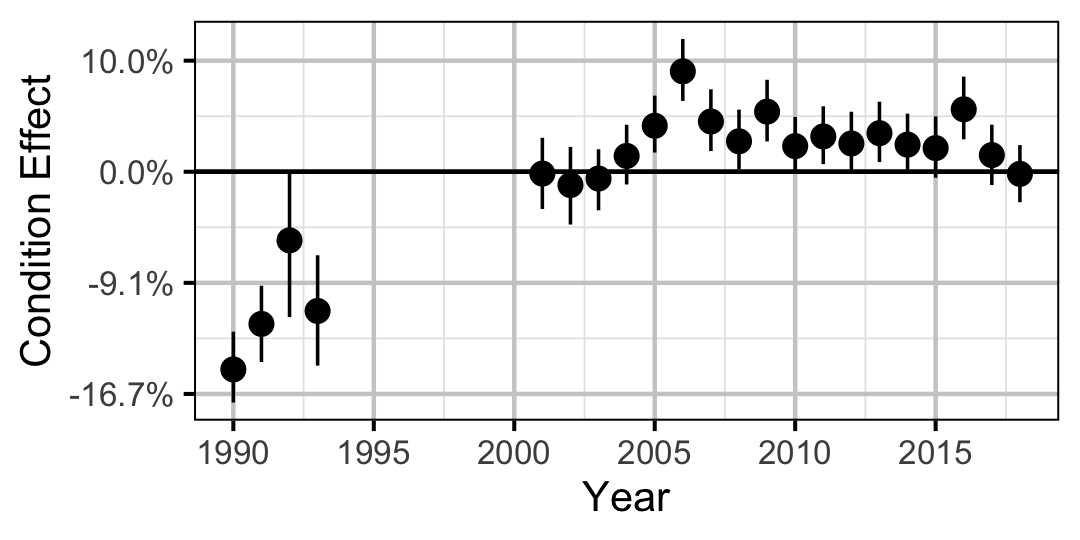Figure 4. Estimated change in condition relative to a typical year for a 350 mm Mountain Whitefish by year (with 95% CRIs).
###### Rainbow Trout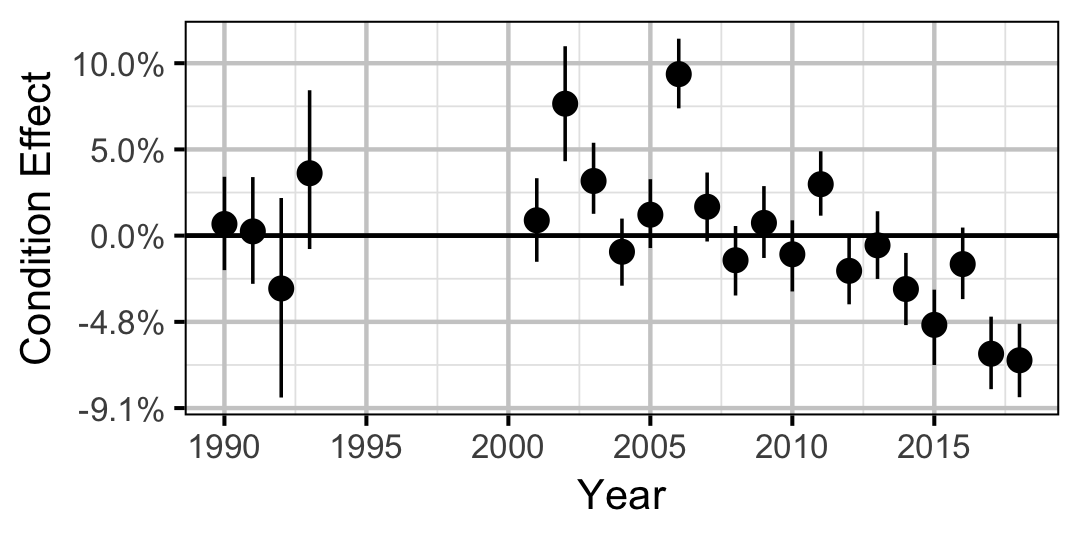Figure 5. Estimated change in condition relative to a typical year for a 500 mm Rainbow Trout by year (with 95% CRIs).
###### Walleye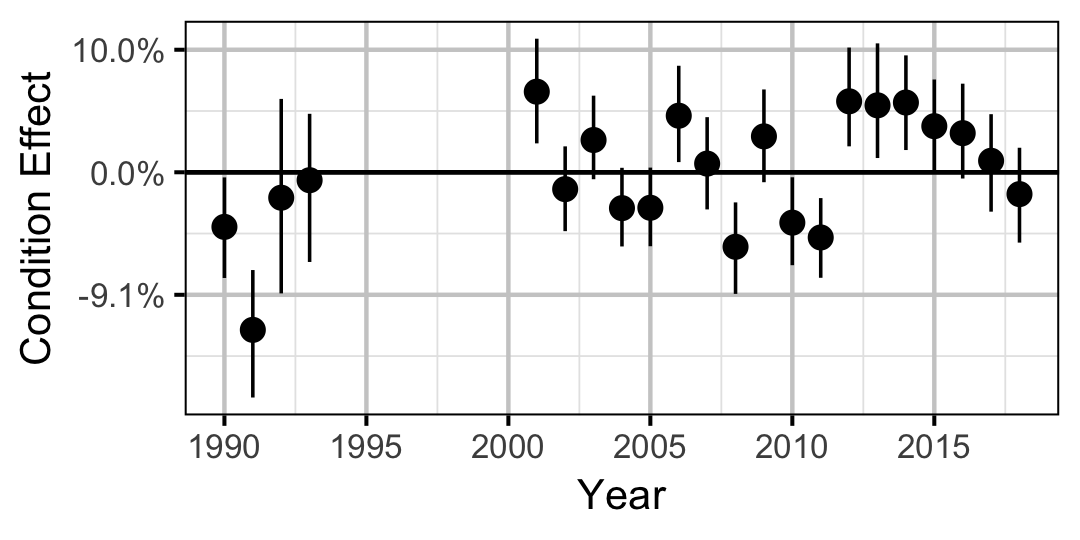Figure 6. Estimated change in condition relative to a typical year for a 600 mm Walleye by year (with 95% CRIs).

#### Growth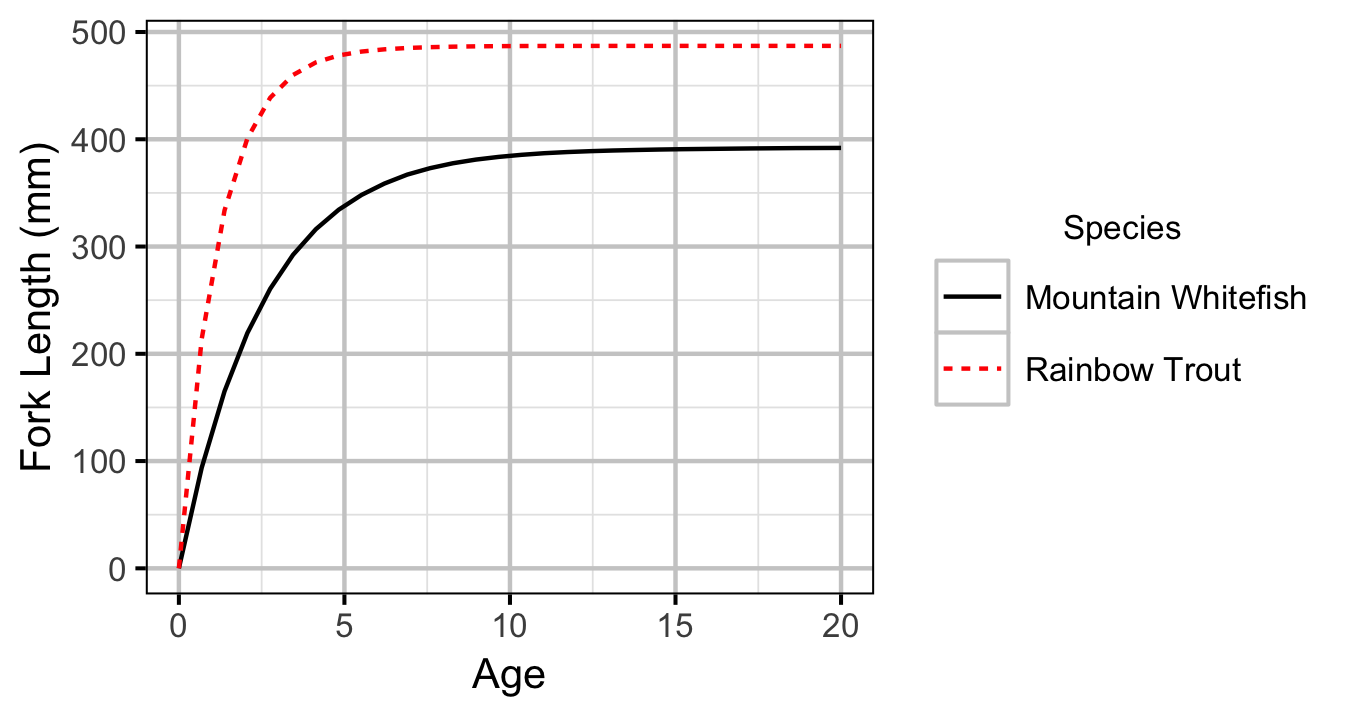Figure 7. Estimated von Bertalanffy growth curve by species. The growth curve for Walleye is not shown because the growth parameters were not representative for the younger age-classes.
##### Mountain Whitefish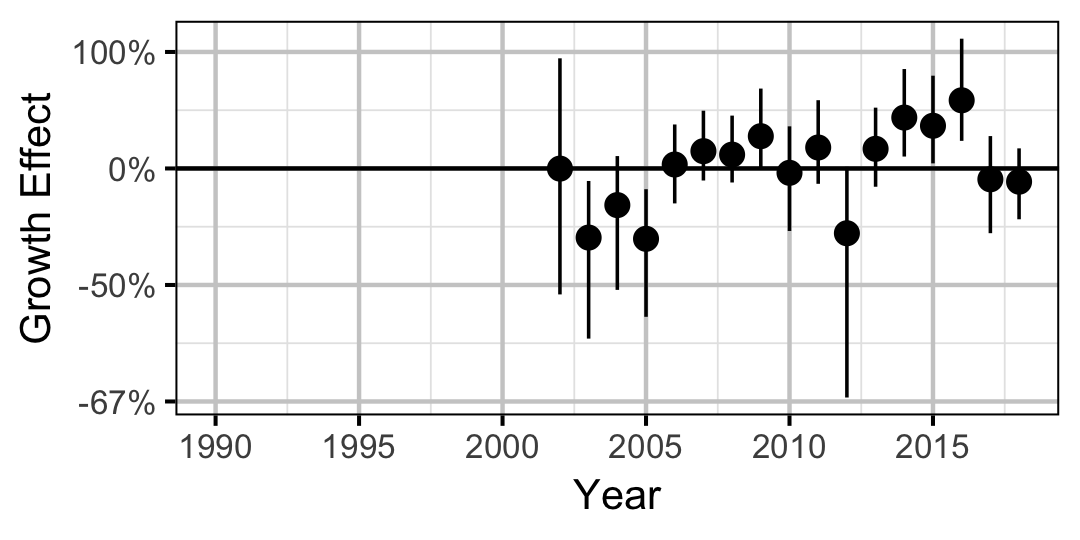Figure 8. Estimated change in von Bertalanffy growth coefficient (k) relative to a typical year by year (with 95% CIs).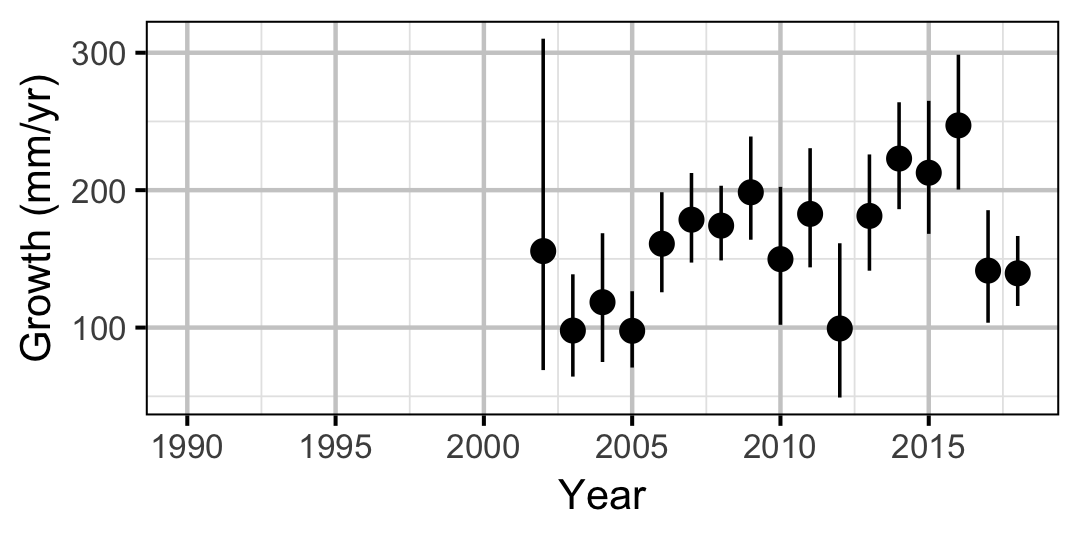Figure 9. Predicted maximum growth by year (with 95% CIs).
##### Rainbow Trout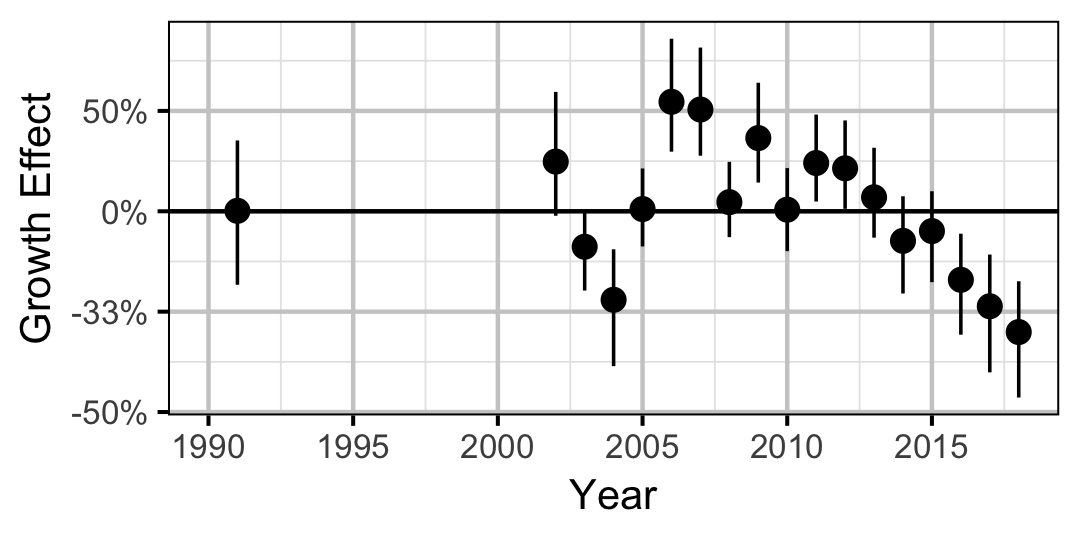Figure 10. Estimated change in von Bertalanffy growth coefficient (k) relative to a typical year by year (with 95% CIs).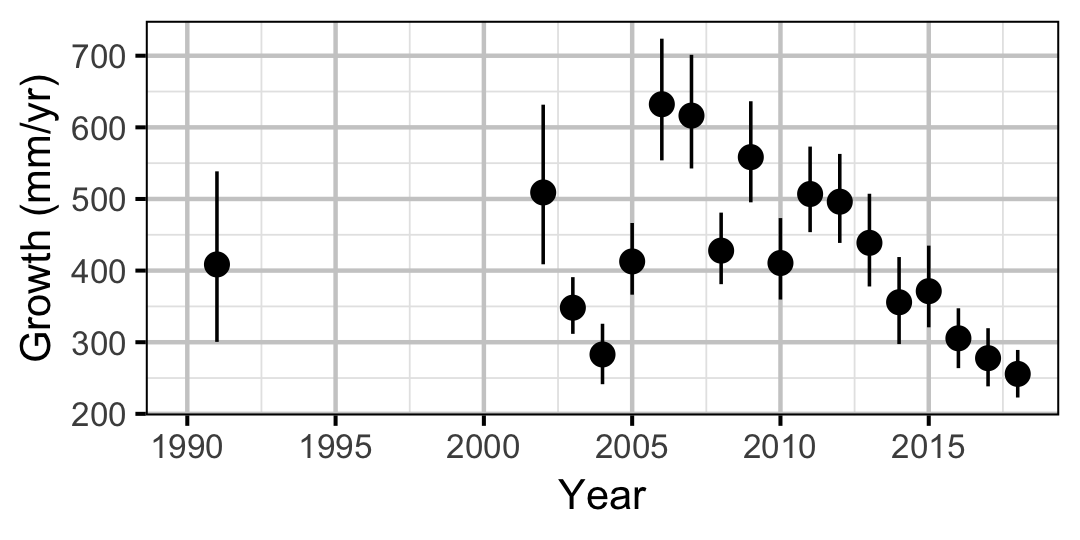Figure 11. Predicted maximum growth by year (with 95% CIs).
##### Walleye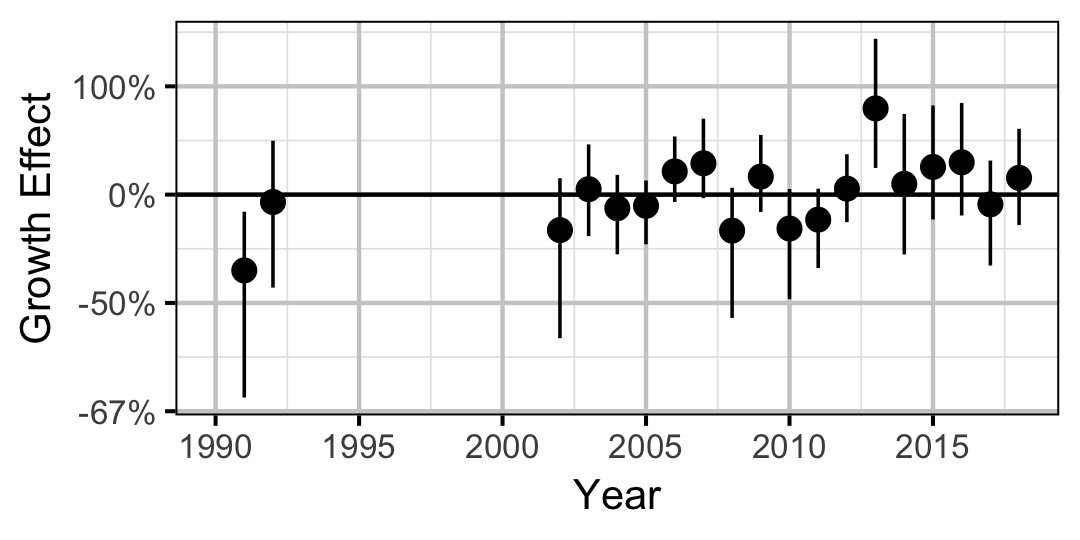Figure 12. Estimated change in von Bertalanffy growth coefficient (k) relative to a typical year by year (with 95% CIs).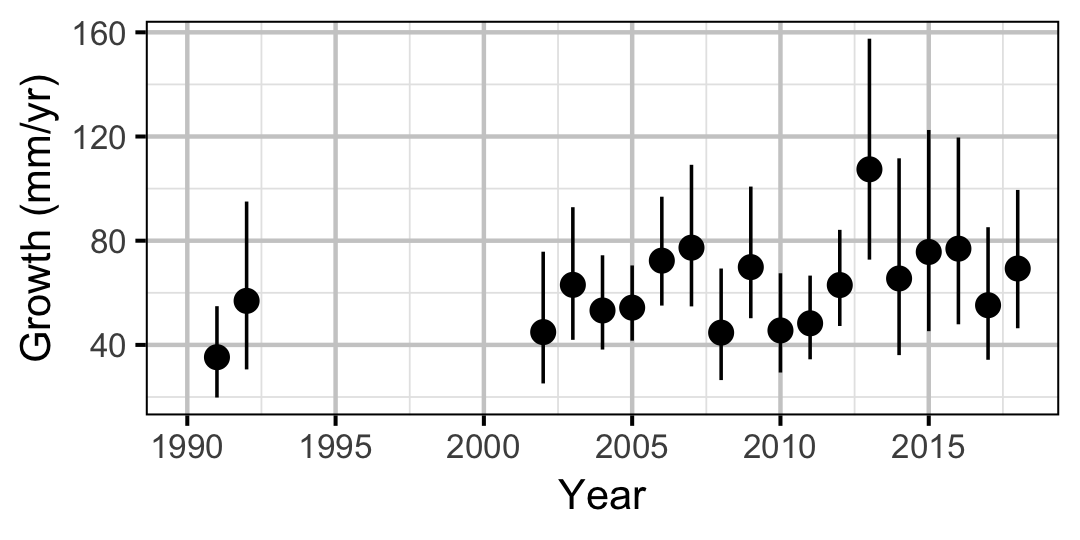Figure 13. Predicted maximum growth by year (with 95% CIs).

#### Movement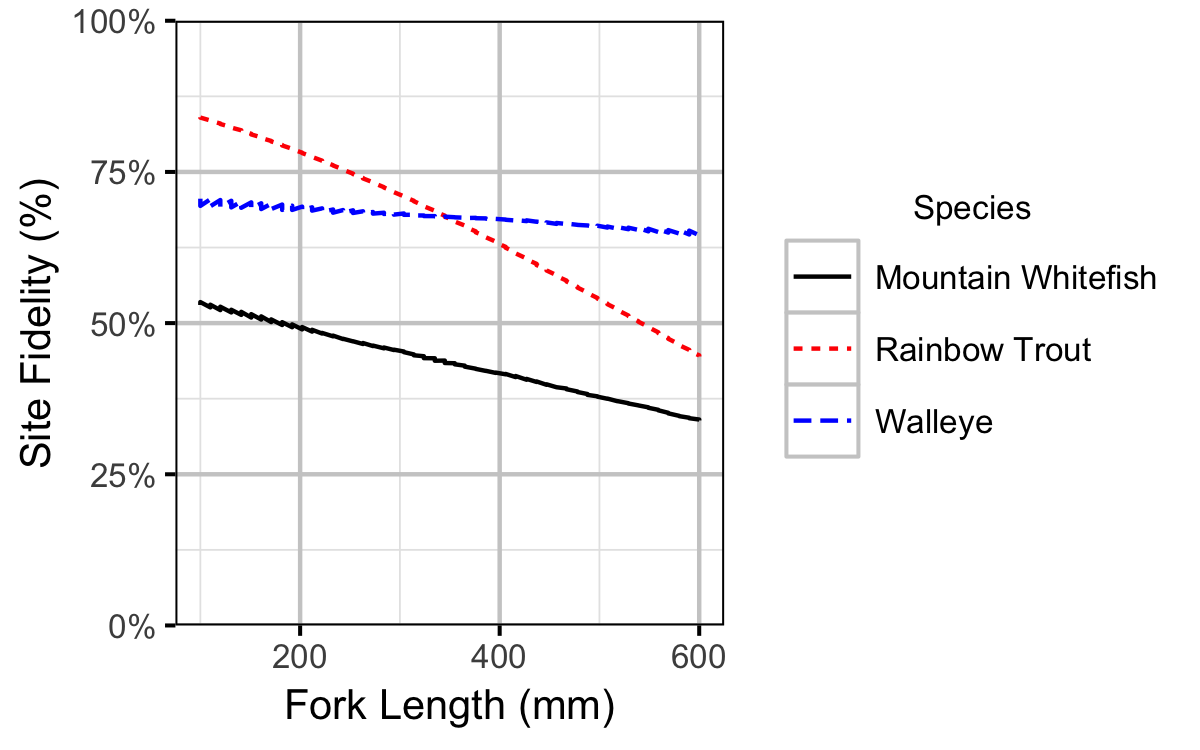Figure 14. Probability of recapture at the same site versus a different site by fish length (with 95% CRIs).

#### Observer Length Correction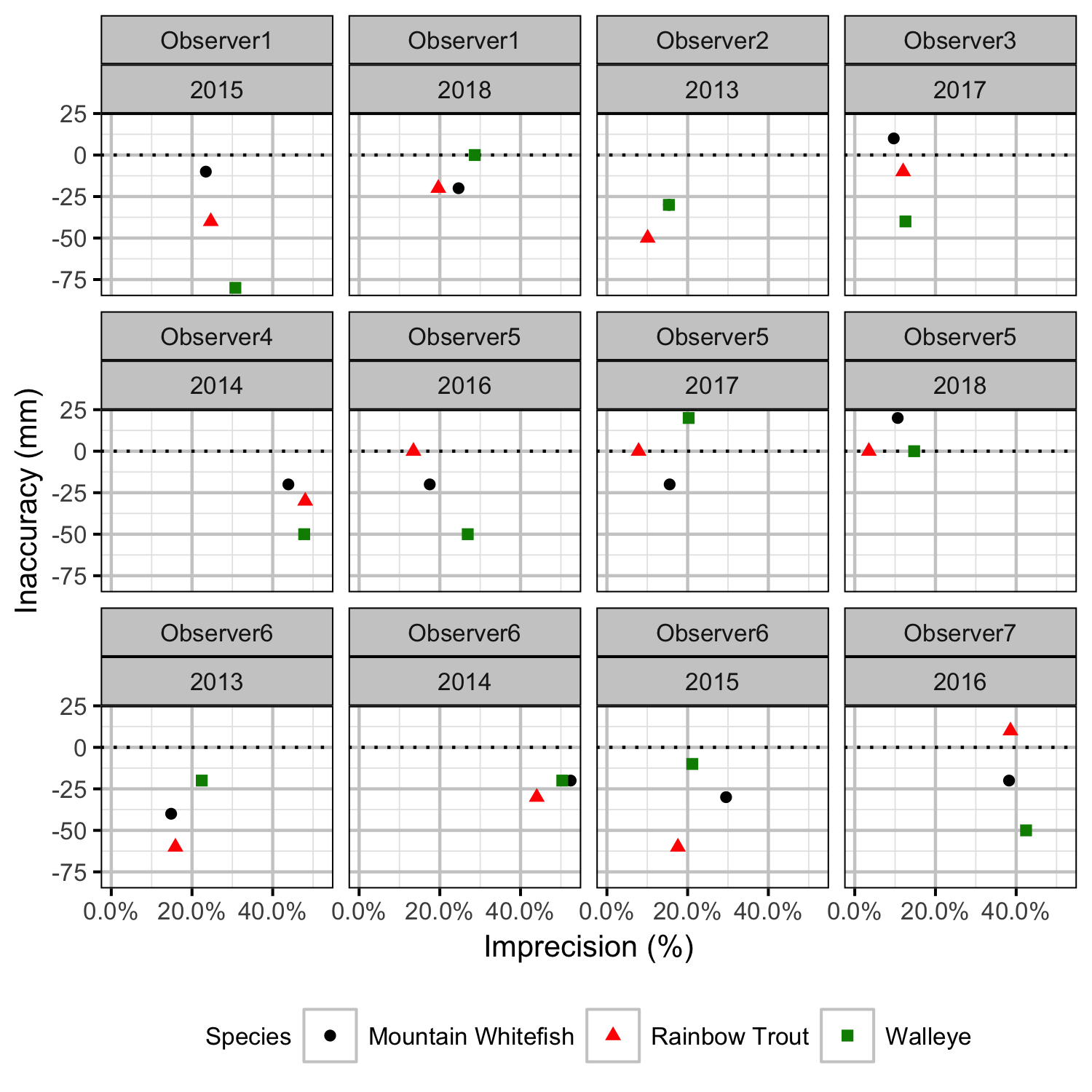Figure 27. Length inaccuracy and imprecision by observer, year and species.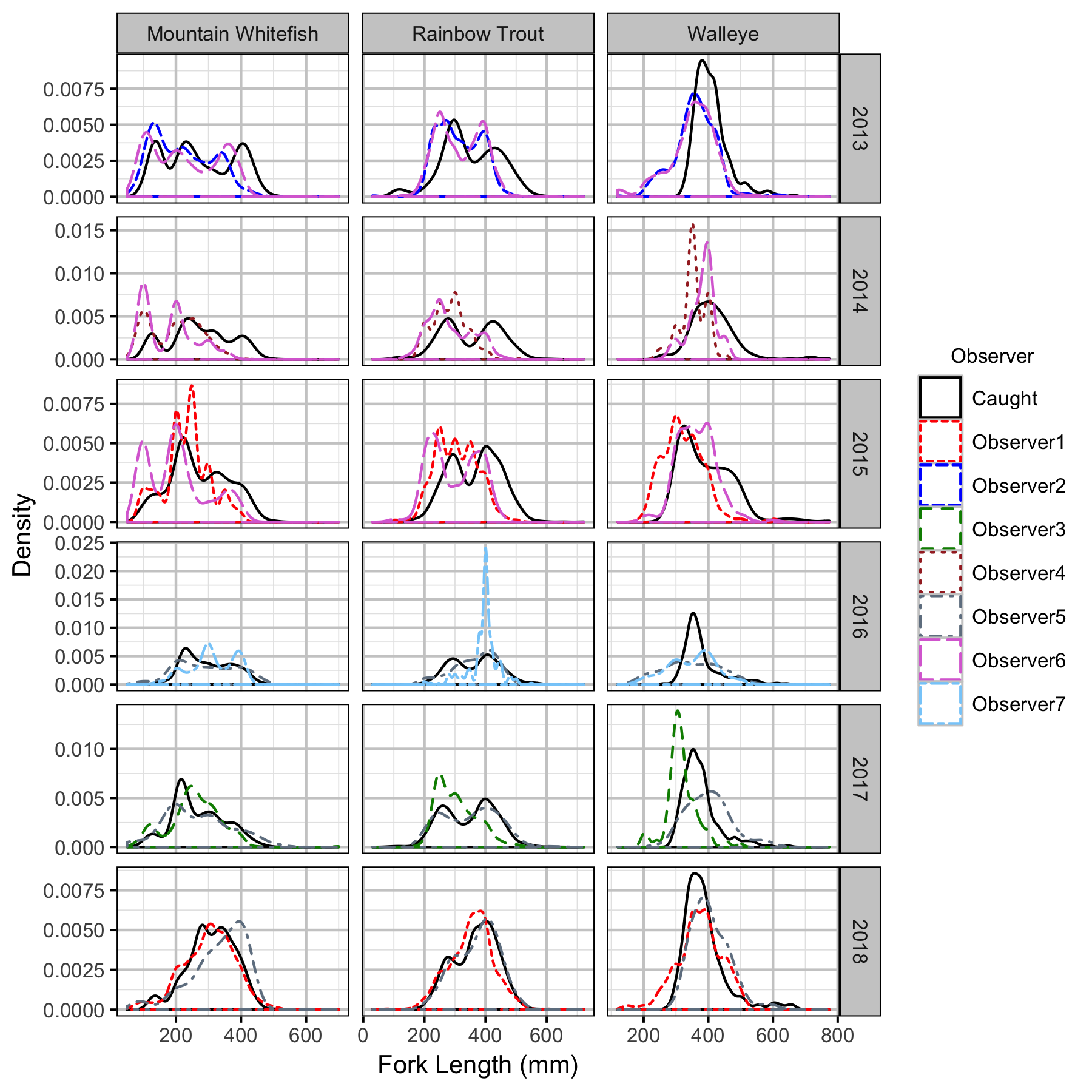Figure 28. Uncorrected length density plots by species, year and observer.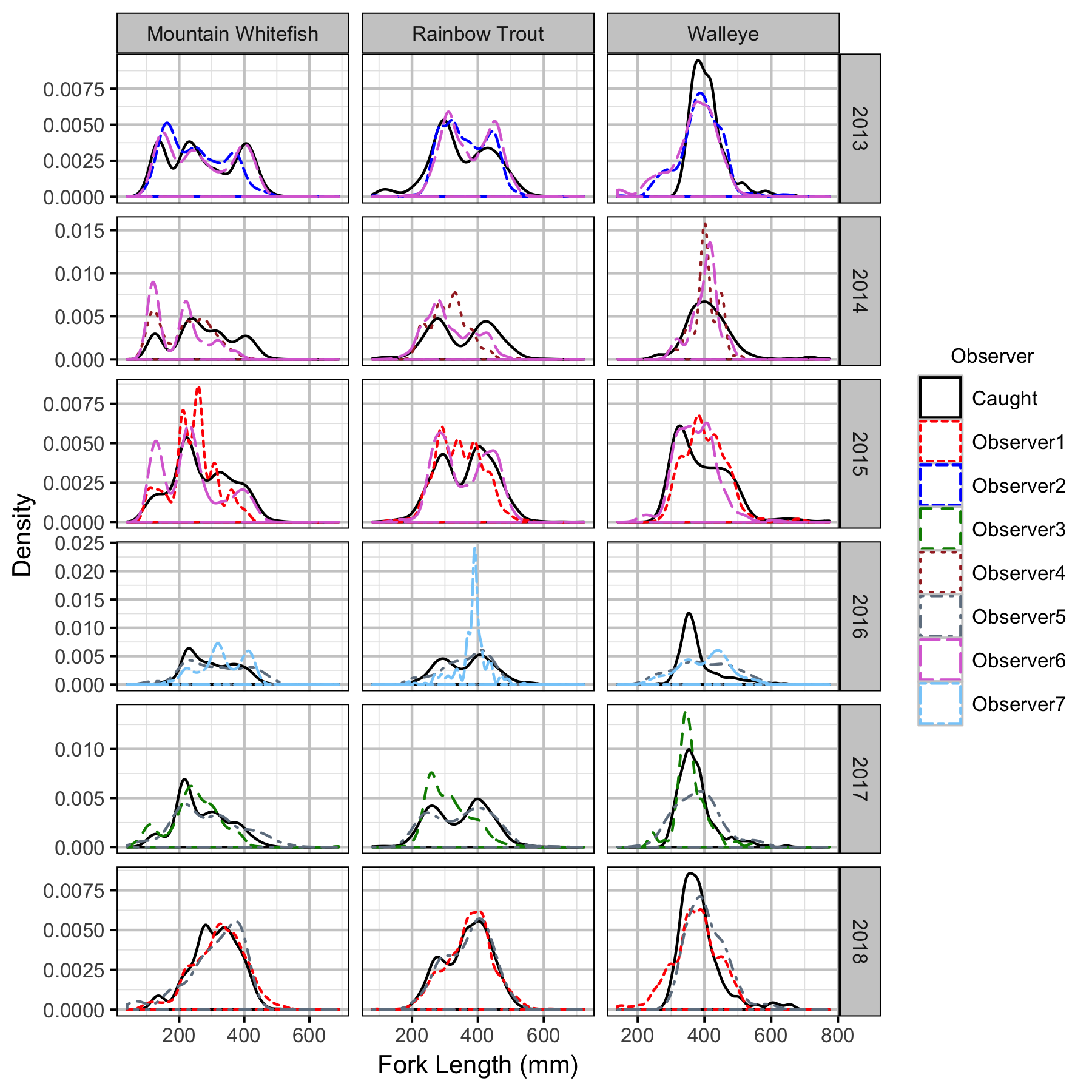Figure 29. Corrected length density plots by species, year and observer.

#### Capture Efficiency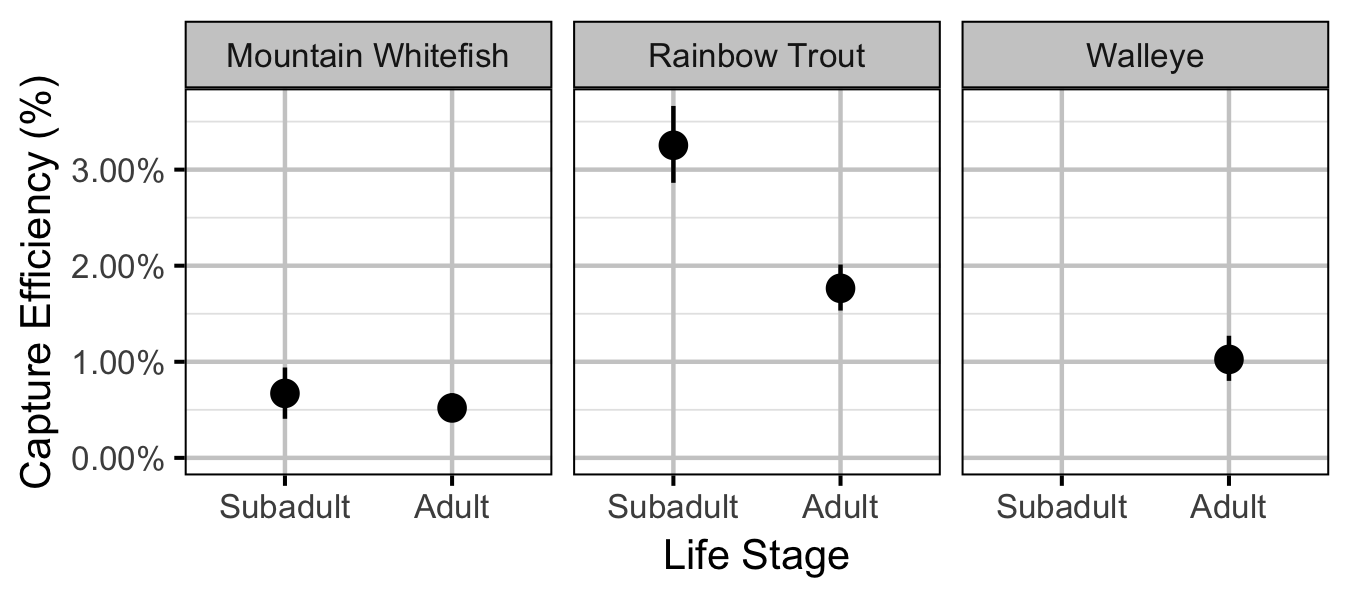Figure 30. Predicted capture efficiency by species and life stage (with 95% CRIs).

#### Abundance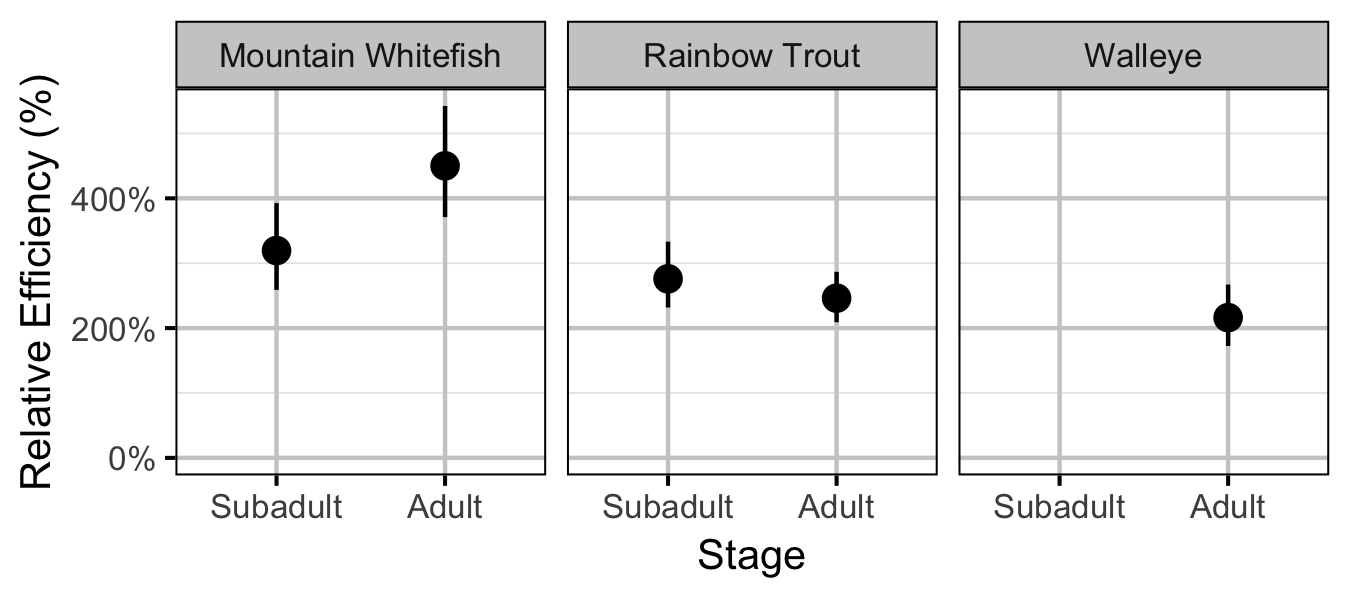Figure 36. Effect of counting (versus capture) on encounter efficiency by species and stage (with 95% CIs).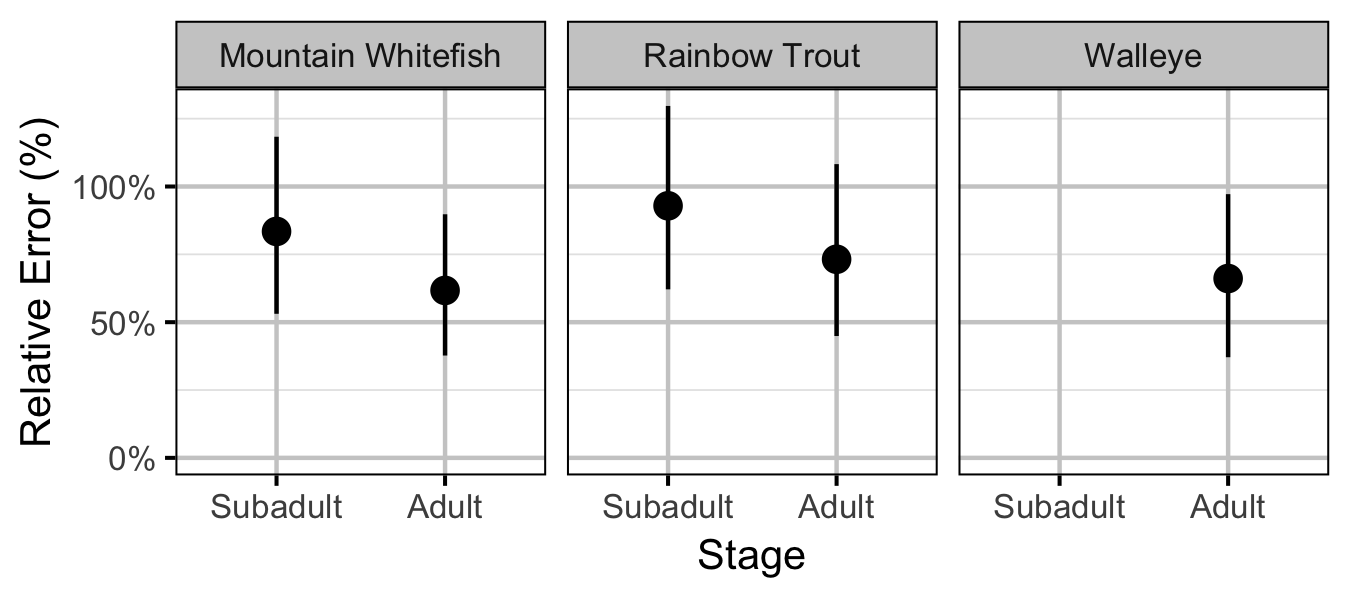Figure 37. Effect of counting (versus capture) on overdispersion by species and stage (with 95% CIs).

#### Stock-Recruitment

##### Mountain Whitefish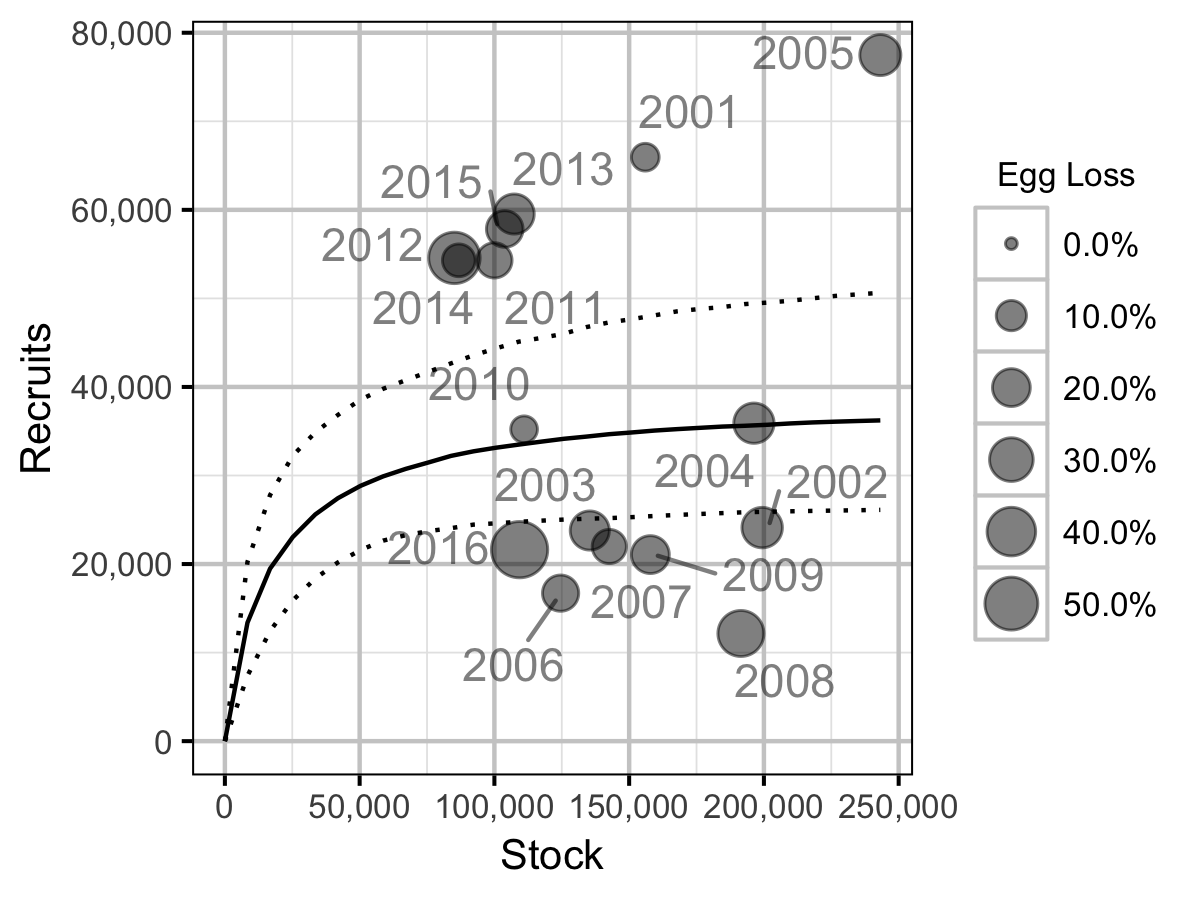Figure 48. Predicted stock-recruitment relationship from Age-2+ spawners to subsequent Age-1 recruits by spawn year (with 95% CRIs).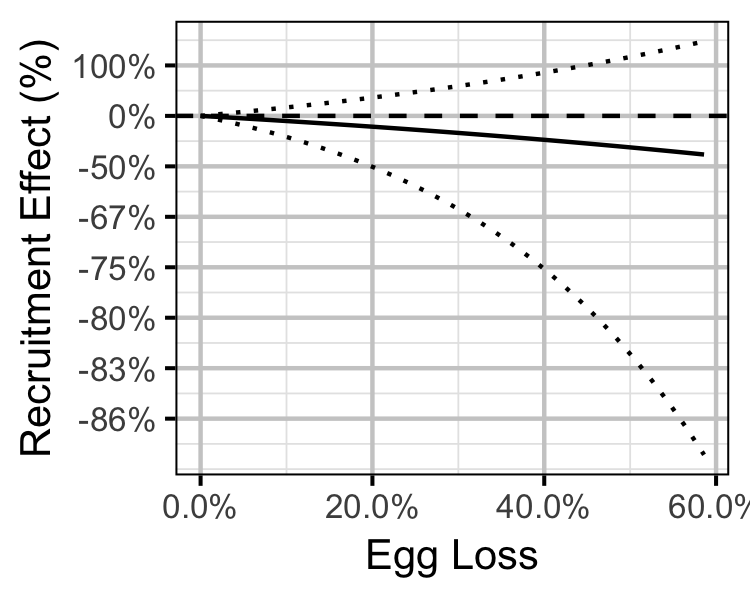Figure 49. Predicted Age-1 recruitment effect by egg loss (with 95% CRIs).
##### Rainbow Trout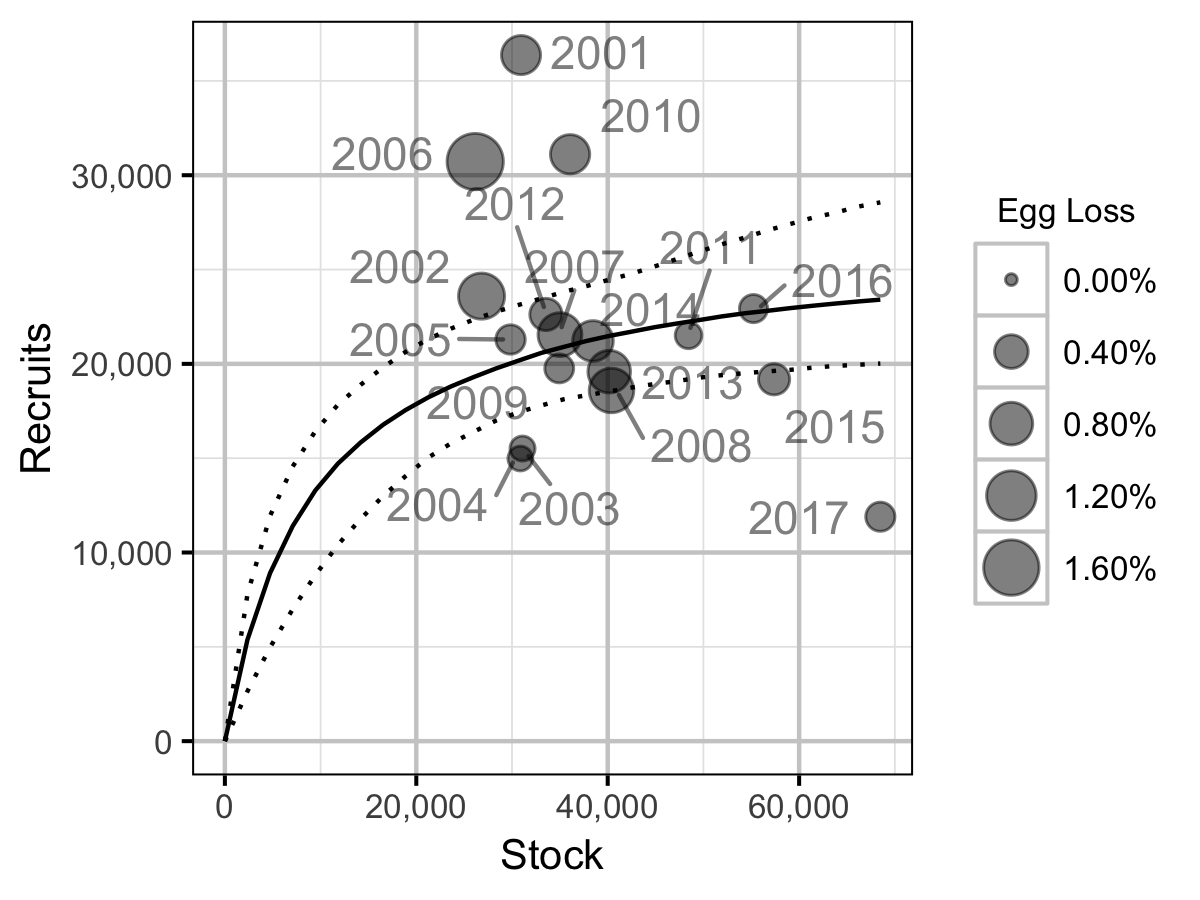Figure 50. Predicted stock-recruitment relationship from Age-2+ spawners to subsequent Age-1 recruits by spawn year (with 95% CRIs).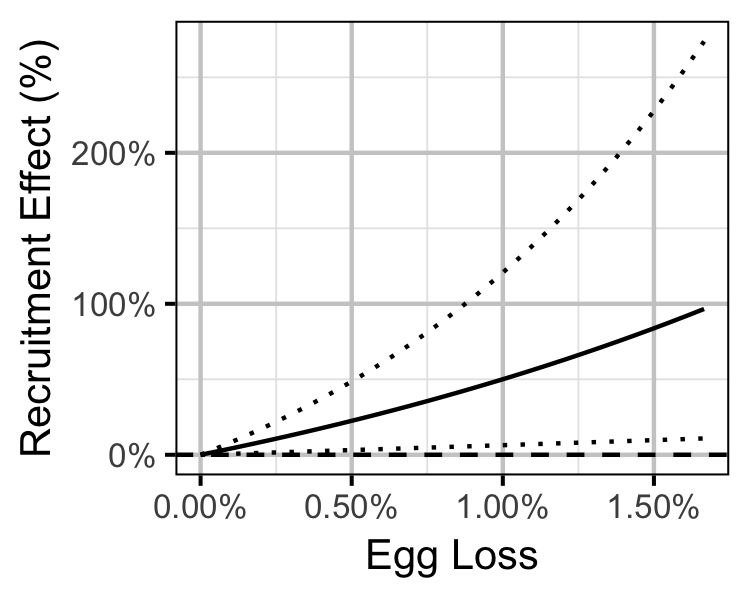Figure 51. Predicted Age-1 recruitment effect by egg loss (with 95% CRIs).

#### Age-Ratios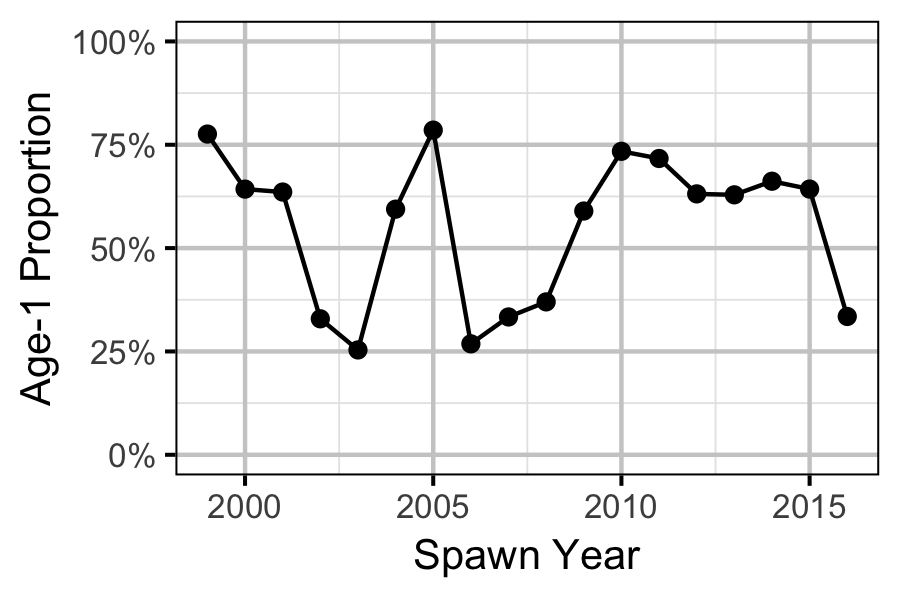Figure 52. Proportion of Age-1 Mountain Whitefish by spawn year.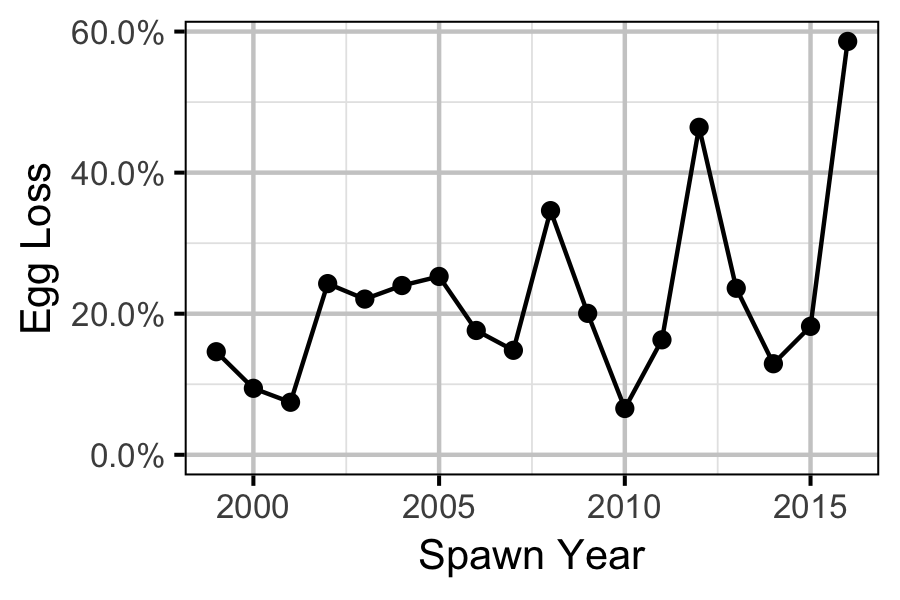Figure 53. Percentage Mountain Whitefish egg loss by spawn year.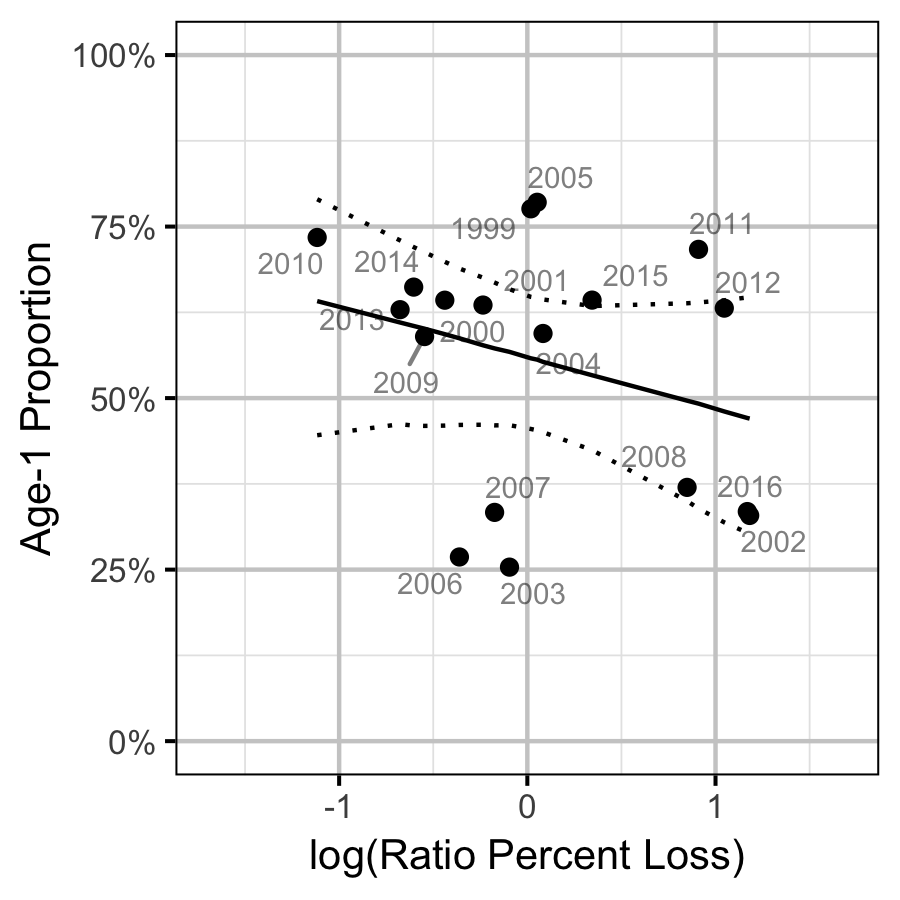Figure 54. Proportion of Age-1 Mountain Whitefish by percentage egg loss ratio, labelled by spawn year. The predicted relationship is indicated by the solid black line (with 95% CRIs).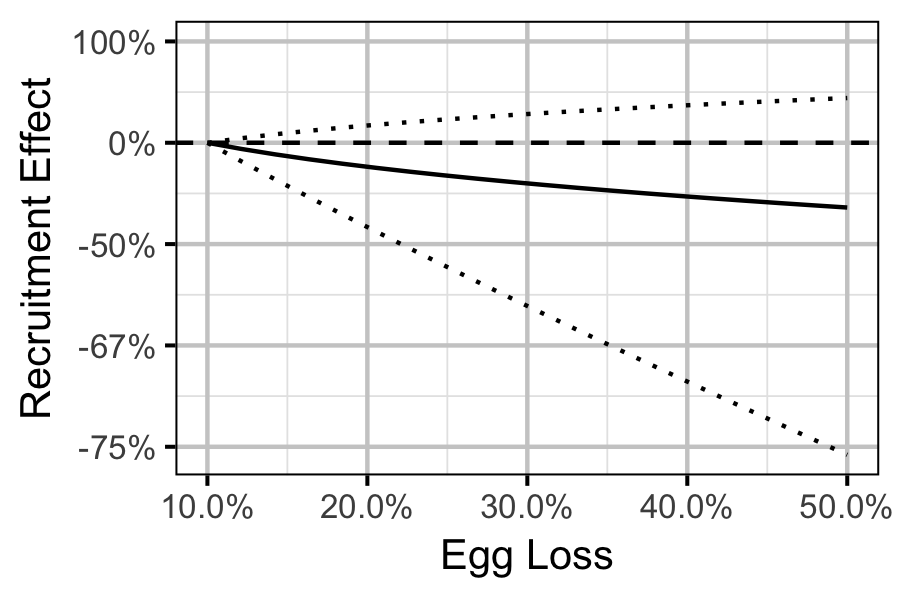Figure 55. Predicted percent change in Age-1 Mountain Whitefish abundance by egg loss in the spawn year relative to 10% egg loss in the spawn year (with 95% CRIs).

## Acknowledgements

The organisations and individuals whose contributions have made this analysis report possible include: